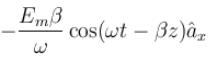Courses

# Propagation Of Em Wave And Gauge Transformation MCQ Level - 2

## 10 Questions MCQ Test Topic wise Tests for IIT JAM Physics | Propagation Of Em Wave And Gauge Transformation MCQ Level - 2

Description
This mock test of Propagation Of Em Wave And Gauge Transformation MCQ Level - 2 for Physics helps you for every Physics entrance exam. This contains 10 Multiple Choice Questions for Physics Propagation Of Em Wave And Gauge Transformation MCQ Level - 2 (mcq) to study with solutions a complete question bank. The solved questions answers in this Propagation Of Em Wave And Gauge Transformation MCQ Level - 2 quiz give you a good mix of easy questions and tough questions. Physics students definitely take this Propagation Of Em Wave And Gauge Transformation MCQ Level - 2 exercise for a better result in the exam. You can find other Propagation Of Em Wave And Gauge Transformation MCQ Level - 2 extra questions, long questions & short questions for Physics on EduRev as well by searching above.
QUESTION: 1

### In a non-conducting medium characterized by ε = ε0 , μ = μ0 and conductivity  the electric field (in V/m) is given by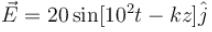The magnetic field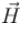(in A/m), is given by

Solution: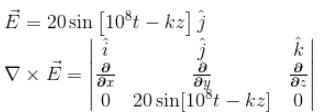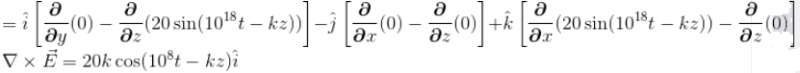We known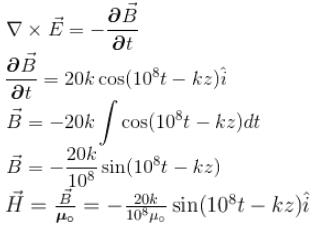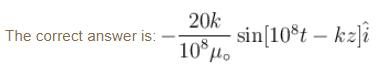QUESTION: 2

### Two conducting plates of infinite extent, one plate at z = 0 and other at z = 1, both parallel to xy-plane. Then the vector and scalar potential in the region between the plate is given by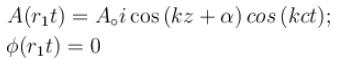The energy density at z = 0, t = 0 and α = π/2

Solution: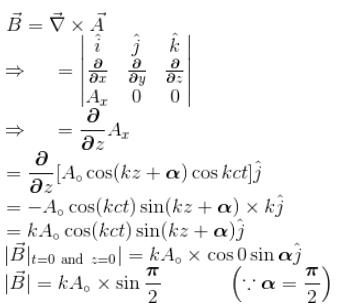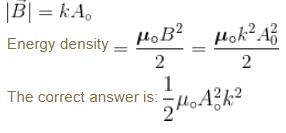QUESTION: 3

### Which one of the following current densities,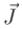can generate the magnetic vector potential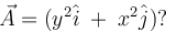Solution:

We know that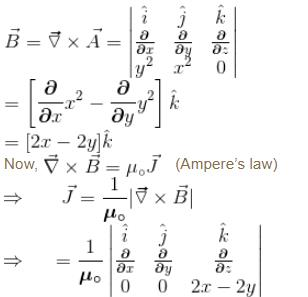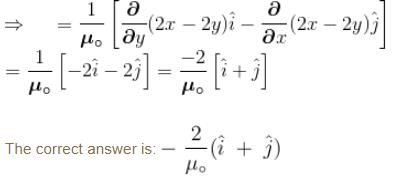QUESTION: 4

The amplitudes of electric and magnetic fields are related to each other by the relation

Solution:

Maxwell’s equation in free space are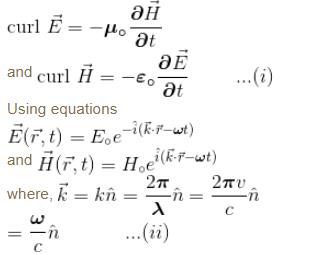Eq. (i) becomes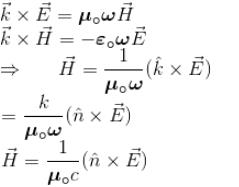This equation in term of modulo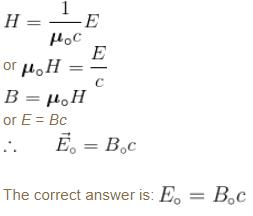QUESTION: 5

A plane electromagnetic wave E2 = 100cos(6 × 108t + 4x)V/m propagates in a medium. Find the value of dielectric constant of the medium.

Solution:

We have E2 = 100 cos (6 × 108t + 4x)                 ....(i)
Comparing this with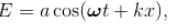we get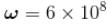....(ii)
k = 4        ....(iii)
Velocity of emf is given = ω/k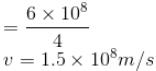By Eq. (iii) refractive index of the medium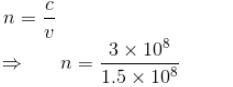⇒ n = 2     ...(v)
The dielectric constant k = (n2)
k = (2)2
k = 4.0

QUESTION: 6

Which of the following expressions for a vector potential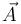does not represent a uniform magnetic field of magnitude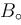along the z-direction?

Solution:

Vector Potential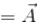So, Magnetic field can be written as.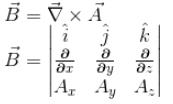⇒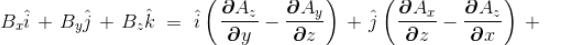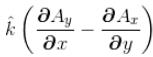∵  B0 is not along z-direction.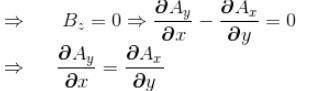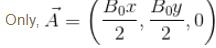satisfies the above condition.
The correct answer is: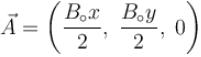QUESTION: 7

An infinitely long hollow cylinder of radius R carrying a surface charge density σ is rotated about its cylindrical axis with a constant angular speed ω. The magnitude of the surface current is

Solution:

Applying Ampere’s law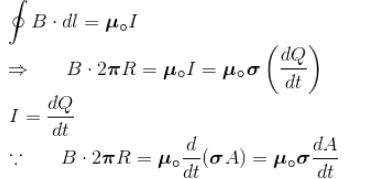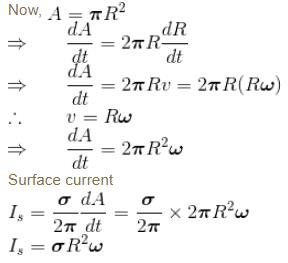QUESTION: 8

In a homogeneous non-conducting region where  μr = 1, the value of εr will be:

Given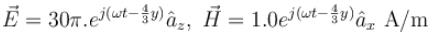Solution: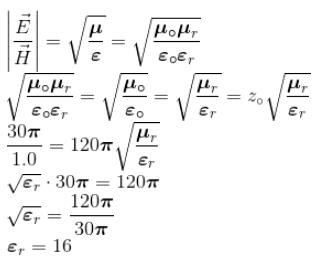QUESTION: 9

The time averaged Poynting vector, in W/m2, for a wave with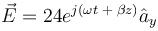in free space

Solution: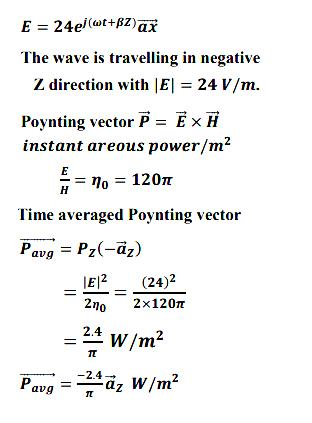QUESTION: 10

Given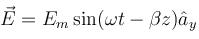in free space, then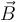will be given as

Solution: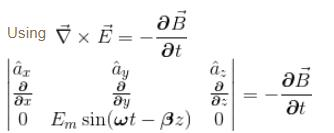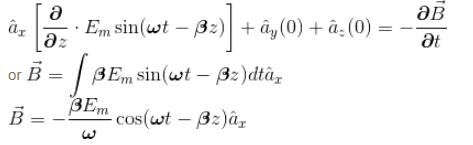The correct answer is: